Percentage Questions And Answers, deals with various concepts which are as under:-

• Convert Percent to Fraction
• Convert Percent to decimal
• Convert Ratio to Percentage
• Convert Fraction to Percent
• Convert Percentage to Ratio
• Finding a number, whose percentage value is given
• Net Increase or Decrease Percentage in a Number
• Finding a number when its decreased percentage is given
• Finding a number when its increased percentage is given

#### Percentage Questions And Answers – Convert Percent to Fraction

Step 1 – In order to convert Percent to Fraction, we divide the Percentage by 100, and remove the sign of Percentage (%).

Step 2 – Simplify the Fraction

Question 1:

Convert 40 % into a Fraction?

Solution:

In order to convert a Percentage into a Fraction, we divide the Percentage by 100, and remove the sign of Percentage (%).

We have,

40 % = 40/100

On Simplifying, 40/100

HCF of 40 and 100 is 20

Divide both 40 and 100 by their HCF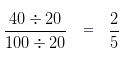#### Percentage Questions And Answers – Convert Percent to decimal

In order to convert Percent to Decimal we have to perform the following steps:

Step 1 – Convert the Percentage into Fraction, by dividing the given Percentage by 100.

Step 2 – Convert the Fraction obtained at Step 1, into a Decimal form , by dividing numerator by Denominator.

Question 2:

Convert 12 % into a decimal.

Solution:

In order to convert given Percentage into into decimal we have to perform the following steps:

Step 1 – Convert the Percentage into Fraction, by dividing the given Percentage by 100

i.e, 12 % = 12/100

Step 2 – Convert the Fraction obtained at Step 1, into a Decimal form , by dividing numerator by Denominator

i.e, 12/100 = 0.12

Hence, 12 % = 0.12

#### Percentage Questions And Answers – Convert Ratio to Percentage

To convert Ratio to Percentage we have to follow following steps:

Step 1 – Convert the Ratio into Fraction.

Step 2  Multiply the Fraction by 100 and put the Percentage sign to the result (%)

Question 3:

Convert 8 : 40 into a Percentage.

Solution:

To convert the given ratio into Percentage we have to follow following steps:

Step 1 – Convert the ratio into Fraction

8 : 40 = 8/40

Step 2 – Multiply the Fraction by 100 and put the Percentage sign to the result (%)

( 8/40 x 100 ) %

On solving the given Fraction, we get,

= 20 %

#### Percentage Questions And Answers – Convert Fraction to Percent

To convert Fraction to Percent, we need to multiply the Fraction by 100, and assign the Percentage (%) sign to it.

Question 4:

Convert 23/100 into Percentage.

Solution:

To convert a Fraction into Percentage, we need to multiply the Fraction by 100, and assign the Percentage (%) sign to it.

that is, ( 23/100 x 100 ) % = 23 %

#### Percentage Questions And Answers – Convert Percentage to Ratio

Step I – In order Convert Percentage to Ratio, we need to divide the given percentage by 100.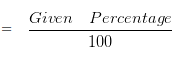Step II  – Reduce the Fraction obtained in Step I into its Simplest form.

Step III – Convert the Fraction ( obtained in step II ) into Ratio by replacing the slash mark with a colon.

Question 5:

Express 8 % as a ratio.

Solution:

In order to express a Percentage as a ratio, we need to write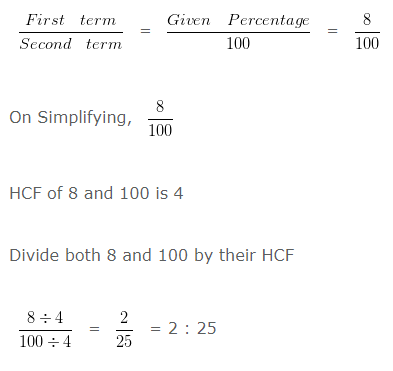#### Percentage Questions And Answers – Finding a number, whose percentage value is given

Question 6:

If 35 % of a number is 105, find the number?

Solution:

We can form a linear equation to express the given statement and then arrive at the number

Let the required number be x

We are given that

35 % of x is 105

or in other words

35/100 × x = 105

On cross multiplication, we get

x = (105×100)/35

x = 300

Hence, the number is 300

#### Percentage Questions And Answers – Net Increase or Decrease Percentage in a Number

Question 7:

A number is increased by 20 % and then decreased by 15 %. The net increase or decrease percentage is?

Solution:

We can form a linear equation to express the given statement and then arrive at the number

Let the required number be “a”

Step I:

If the number is increased by 20 % it would be equal to ( Given Number + 20 % of Given Number )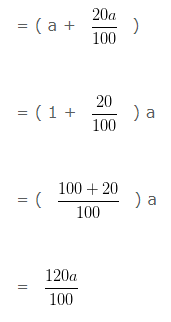Step II:

If the number at Step 1 is decreased by 15 % it would be equal to ( Increased Number – 15 % of Increased Number )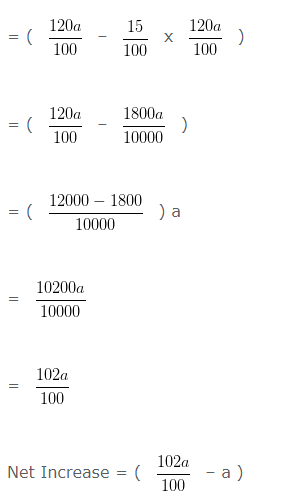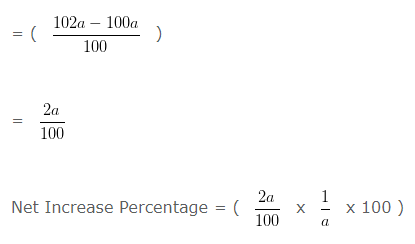= 2 %

Hence, Net Increase Percentage is = 2 %

#### Percentage Questions And Answers – Finding a number when its decreased percentage is given

Question 8:

A number when decreased by 15 % gives 170. The number is?

Solution:

Here we take the help of linear equation. First we will form the equation.

Let the number be ‘a’

15 % of ‘a’ = (15/100)a

Since, the equation is:

a – (15/100)a = 170

Taking 100 as LCM on LHS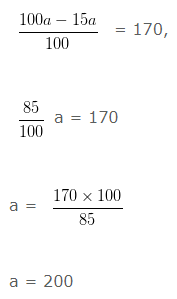Hence, the number is 200

#### Percentage Questions And Answers – Finding a number when its increased percentage is given

Question 9:

A number, when increased by 50% gives 150. The number is?

Solution:

The problem can be solved using linear equation.

Let the number be ‘a’

50% of a = (50/100)a

We are given that, a + (50/100)a = 150

Taking 100 as LCM on LHS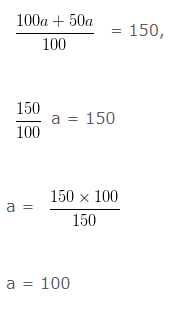Hence, the number is 100